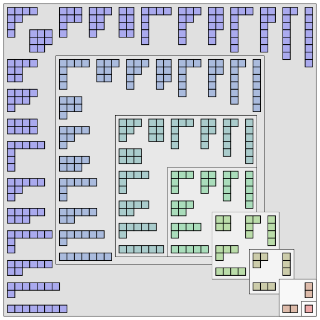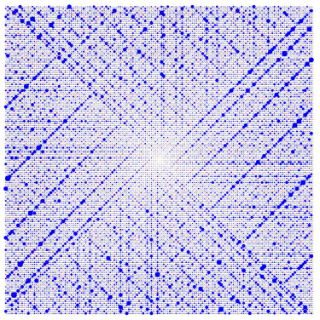# Partition

Topic | v1 | created by jjones |Description

In number theory and combinatorics, a partition of a positive integer n, also called an integer partition, is a way of writing n as a sum of positive integers. Two sums that differ only in the order of their summands are considered the same partition. (If order matters, the sum becomes a composition.) For example, 4 can be partitioned in five distinct ways: 4 3 + 1 2 + 2 2 + 1 + 1 1 + 1 + 1 + 1 The order-dependent composition 1 + 3 is the same partition as 3 + 1, while the two distinct compositions 1 + 2 + 1 and 1 + 1 + 2 represent the same partition 2 + 1 + 1. A summand in a partition is also called a part. The number of partitions of n is given by the partition function p(n). So p(4) = 5. The notation λ ⊢ n means that λ is a partition of n. Partitions can be graphically visualized with Young diagrams or Ferrers diagrams.

##### Relations

Number theory (or arithmetic or higher arithmetic in older usage) is a branch of pure mathematics dev...##### Resources

10.0 rating 3.0 level 9.0 clarity 3.0 background – 1 rating

This video is about one or my all-time favourite theorems in math(s): Euler's amazing pentagonal numb...